Next: Resonances Up: Waves in Cold Plasmas Previous: Wave Propagation Through Inhomogeneous

# Cutoffs

We have seen that electromagnetic wave propagation (in one dimension) through an inhomogeneous plasma, in the physically relevant limit in which the variation length-scale of the plasma is much greater than the wave-length of the wave, is well described by the WKB solutions, (569)-(570). However, these solutions break down in the immediate vicinity of a cutoff, where, or a resonance, where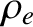. Let us now examine what happens to electromagnetic waves propagating through a plasma when they encounter a cutoff or a resonance.

Suppose that a cutoff is located at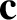, so that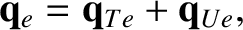(571)

in the immediate vicinity of this point, where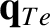. It is evident, from the WKB solutions, (569)-(570), that the cutoff point lies at the boundary between a region (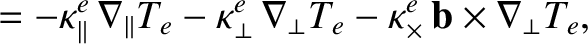) in which electromagnetic waves propagate, and a region (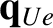) in which the waves are evanescent. In a physically realistic solution, we would expect the wave amplitude to decay (as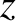decreases) in the evanescent region. Let us search for such a wave solution.

In the immediate vicinity of the cutoff point,, Eqs. (555) and (571) yield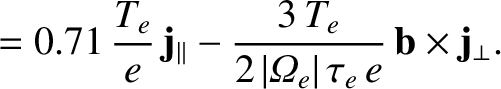(572)

where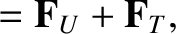(573)

Equation (572) is a standard equation, known as Airy's equation, and possesses two independent solutions, denoted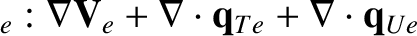and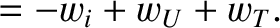.The second solution,, is unphysical, since it blows up as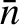. The physical solution,, has the asymptotic behaviour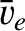(574)

in the limit, and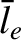(575)

in the limit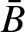.

Suppose that a unit amplitude plane electromagnetic wave, polarized in the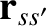-direction, is launched from an antenna, located at large positive, towards the cutoff point at. It is assumed that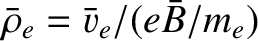at the launch point. In the non-evanescent region,, the wave can be represented as a linear combination of propagating WKB solutions: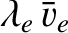(576)

The first term on the right-hand side of the above equation represents the incident wave, whereas the second term represents the reflected wave. The complex constant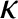is the coefficient of reflection. In the vicinity of the cutoff point (i.e.,small and positive, or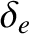large and positive) the above expression reduces to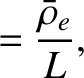(577)

However, we have another expression for the wave in this region. Namely,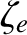(578)

where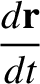is an arbitrary constant. The above equation can be written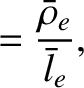(579)

A comparison of Eqs. (577) and (579) yields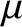(580)

In other words, at a cutoff point there is total reflection, since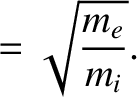, with a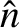phase-shift.Next: Resonances Up: Waves in Cold Plasmas Previous: Wave Propagation Through Inhomogeneous
Richard Fitzpatrick 2011-03-31